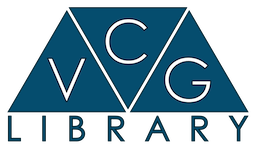\$darkmodeVCG Libraryvcg::tri::UpdateCurvature< MeshType > Class Template Reference

Management, updating and computation of per-vertex and per-face normals. More...

`#include <vcg/complex/algorithms/update/curvature.h>`

## Public Types

typedef MeshType::FaceType FaceType

typedef MeshType::FacePointer FacePointer

typedef MeshType::FaceIterator FaceIterator

typedef MeshType::VertexIterator VertexIterator

typedef MeshType::VertContainer VertContainer

typedef MeshType::VertexType VertexType

typedef MeshType::VertexPointer VertexPointer

typedef vcg::face::VFIterator< FaceType > VFIteratorType

typedef MeshType::CoordType CoordType

typedef CoordType::ScalarType ScalarType

typedef MeshType::VertexType::CurScalarType CurScalarType

typedef MeshType::VertexType::CurVecType CurVecType

typedef vcg::GridStaticPtr< FaceType, ScalarType > MeshGridType

typedef vcg::GridStaticPtr< VertexType, ScalarType > PointsGridType

## Static Public Member Functions

static void PrincipalDirections (MeshType &m)
Compute principal direction and magnitudo of curvature.

static void PrincipalDirectionsPCA (MeshType &m, ScalarType r, bool pointVSfaceInt=true, vcg::CallBackPos *cb=NULL)

static void MeanAndGaussian (MeshType &m)
Computes the discrete mean gaussian curvature. More...

static void PerVertexAbsoluteMeanAndGaussian (MeshType &m)
Update the mean and the gaussian curvature of a vertex. More...

static void PrincipalDirectionsNormalCycle (MeshType &m)

static void PerVertexBasicRadialCrossField (MeshType &m, float anisotropyRatio=1.0)

## Detailed Description

### template<class MeshType> class vcg::tri::UpdateCurvature< MeshType >

Management, updating and computation of per-vertex and per-face normals.

This class is used to compute or update the normals that can be stored in the vertex or face component of a mesh.

## ◆ MeshGridType

template<class MeshType >
 typedef vcg::GridStaticPtr vcg::tri::UpdateCurvature< MeshType >::MeshGridType

Curvature meseaure as described in the paper: Robust principal curvatures on Multiple Scales, Yong-Liang Yang, Yu-Kun Lai, Shi-Min Hu Helmut Pottmann SGP 2004 If pointVSfaceInt==true the covariance is computed by montecarlo sampling on the mesh (faster) If pointVSfaceInt==false the covariance is computed by (analytic)integration over the surface (slower)

## ◆ MeanAndGaussian()

template<class MeshType >
 static void vcg::tri::UpdateCurvature< MeshType >::MeanAndGaussian ( MeshType & m )
inlinestatic

Computes the discrete mean gaussian curvature.

The algorithm used is the one Desbrun et al. that is based on a discrete analysis of the angles of the faces around a vertex.

For further details, please, refer to:
Discrete Differential-Geometry Operators for Triangulated 2-Manifolds
Mark Meyer, Mathieu Desbrun, Peter Schroder, Alan H. Barr
VisMath '02, Berlin

## ◆ PerVertexAbsoluteMeanAndGaussian()

template<class MeshType >
 static void vcg::tri::UpdateCurvature< MeshType >::PerVertexAbsoluteMeanAndGaussian ( MeshType & m )
inlinestatic

Update the mean and the gaussian curvature of a vertex.

The function uses the VF adiacency to walk around the vertex.

Based on the paper "Optimizing 3d triangulations using discrete curvature analysis" it compute an approximation of the gaussian and of the absolute mean curvature

The documentation for this class was generated from the following file:
• /home/cignoni/devel/vcglib/vcg/complex/algorithms/update/curvature.h